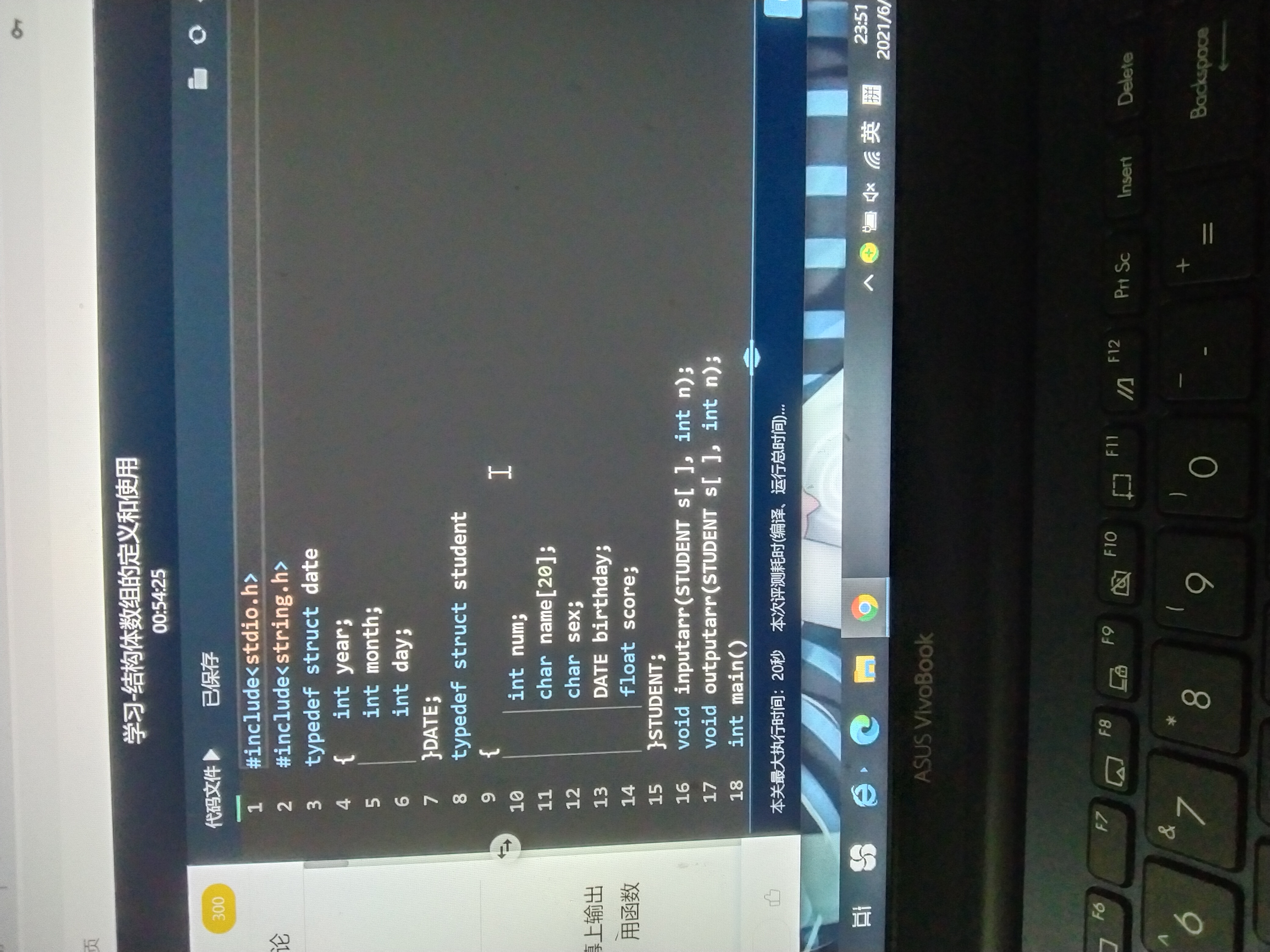2021-06-04 23:53

# 输入5个学生的信息，输出这五个学生的信息，用结构体数组保存学生的信息，实现结构体数组的输入输出。• 写回答
• 好问题 提建议
• 关注问题
• 收藏
• 邀请回答

#### 2条回答默认 最新

•qfl_sdu 2021-06-05 00:06
已采纳

代码如下，如有帮助，请采纳一下，谢谢。

``````#include <stdio.h>
#include <string.h>
typedef struct date
{
int year;
int month;
int day;
}DATE;

typedef struct student
{
int num;
char name;
char sex;
DATE birthday;
float score;
}STUDENT;

void inputarr(STUDENT s[],int n)
{
int i = 0;
printf("请输入%d个学生的学号、姓名、性别、生日、成绩\n",n);
for (; i < n; i++)
{
scanf("%d",&s[i].num);
scanf("%s",s[i].name);
scanf(" %c%*c",&s[i].sex);
scanf("%d %d %d",&s[i].birthday.year,&s[i].birthday.month,&s[i].birthday.day);
scanf("%f",&s[i].score);
}
}

void outputarr(STUDENT s[],int n)
{
int i = 0;
for (; i < n; i++)
{
printf("%d  %s   %c   %d-%d-%d   %.2f\n",s[i].num,s[i].name,s[i].sex,s[i].birthday.year,s[i].birthday.month,s[i].birthday.day,s[i].score);
}
}

int main()
{
STUDENT s;
inputarr(s,3);
outputarr(s,3);
return 0;
}``````
已采纳该答案
评论
解决 无用
打赏 举报
•有问必答小助手 2021-06-07 11:15

您好，我是有问必答小助手，您的问题已经有小伙伴解答了，您看下是否解决，可以追评进行沟通哦~

如果有您比较满意的答案 / 帮您提供解决思路的答案，可以点击【采纳】按钮，给回答的小伙伴一些鼓励哦～～